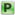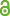Hauptmenü
• Autor
• Berkes, István
• Györfi, László
• Kevei, Péter
• TitelTail probabilities of St. Petersburg sums, trimmed sums, and their limit
• Datei
• DOI10.1007/s10959-016-0677-5
• Erschienen inJournal of Theoretical Probability
• Band30
• Erscheinungsjahr2017
• Heft3
• Seiten1104-1129
• LicenceCC BY
• ISSN1572-9230
• Zugriffsrechte• AbstractWe provide exact asymptotics for the tail probabilities $$\mathbb{P}\{S_n > x\}$$ and $$\mathbb{P}\{S_n − X_n^∗ > x\}$$ as $$x → ∞$$, for fix n, where $$S_n$$ and $$X_n^∗$$ is the partial sum and partial maximum of i.i.d. St. Pe- tersburg random variables. We show that while the order of the tail of the sum $$S_n$$ is $$x^{−1}$$ , the order of the tail of the trimmed sum $$S_n − X_n^∗$$ is $$x^{−2}$$ . In particular, we prove that al- though the St. Petersburg distribution is only O-subexponential, the subexponential property almost holds. We also provide an infinite series representation of the distribution function of the limiting distribution of the trimmed sum, and analyze its tail behavior.